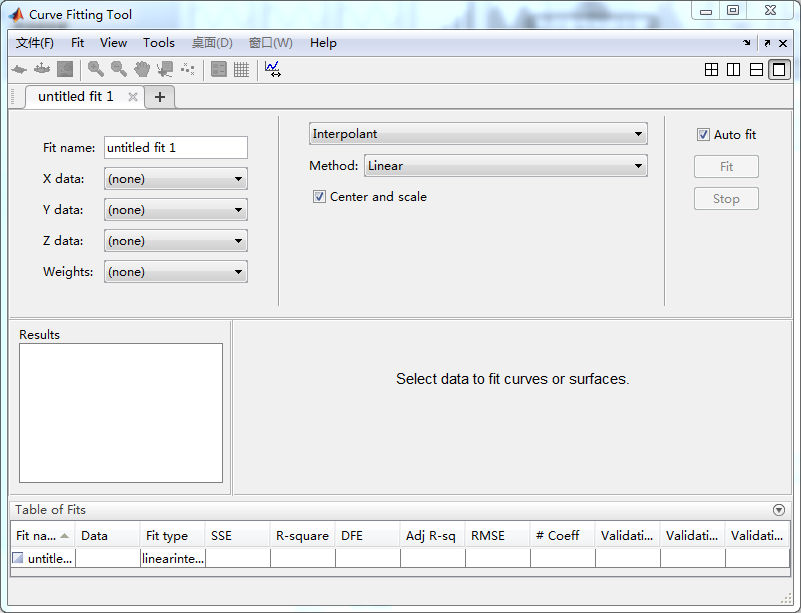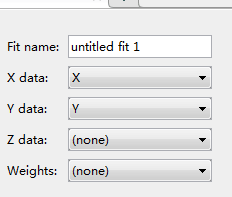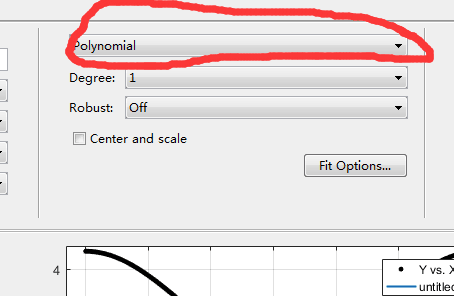• matlab——工具箱使用（线性拟合） 本文目录1. 将linar_ana.m文件运行后，点击App->Curve Fitting(注意版本为R2018b)2. 选择拟合数据![在这里插入图片描述]...
matlab——工具箱使用（线性拟合）

本文目录

1. 将linar_ana.m文件运行后，点击App->Curve Fitting(注意版本为R2018b)

这里数据我没有给出，数据源转至GitHib上查看，链接等我上传以后再放

1. 将linar_ana.m文件运行后，点击App->Curve Fitting(注意版本为R2018b)展开全文• Matlab有一个功能强大的曲线拟合工具箱 cftool ，使用方便，能实现多种类型的线性、非线性曲线拟合。下面结合我使用的 Matlab R2007b 来简单介绍如何使用这个工具箱。 假设我们要拟合的函数形式是 y=A*x*x + B*...
一、 单一变量的曲线逼近 Matlab有一个功能强大的曲线拟合工具箱 cftool ，使用方便，能实现多种类型的线性、非线性曲线拟合。下面结合我使用的 Matlab R2007b 来简单介绍如何使用这个工具箱。  假设我们要拟合的函数形式是 y=A*x*x + B*x, 且A>0,B>0 。  1、在命令行输入数据： 》x=[110.3323 148.7328 178.064 202.8258033 224.7105 244.5711 262.908 280.0447 296.204 311.5475]； 》y=[5 10 15 20 25 30 35 40 45 50]；  2、启动曲线拟合工具箱 》cftool  3、进入曲线拟合工具箱界面“Curve Fitting tool” （1）点击“Data”按钮，弹出“Data”窗口； （2）利用X data和Y data的下拉菜单读入数据x,y，可修改数据集名“Data set name”，然后点击“Create data set”按钮，退出“Data”窗口，返回工具箱界面，这时会自动画出数据集的曲线图； （3）点击“Fitting”按钮，弹出“Fitting”窗口； （4）点击“New fit”按钮，可修改拟合项目名称“Fit name”，通过“Data set”下拉菜单选择数据集，然后通过下拉菜单“Type of fit”选择拟合曲线的类型，工具箱提供的拟合类型有：  Custom Equations：用户自定义的函数类型  Exponential：指数逼近，有2种类型， a*exp(b*x) 、 a*exp(b*x) + c*exp(d*x)  Fourier：傅立叶逼近，有7种类型，基础型是 a0 + a1*cos(x*w) + b1*sin(x*w)  Gaussian：高斯逼近，有8种类型，基础型是 a1*exp(-((x-b1)/c1)^2)  Interpolant：插值逼近，有4种类型，linear、nearest neighbor、cubic spline、shape-preserving  Polynomial：多形式逼近，有9种类型，linear ~、quadratic ~、cubic ~、4-9th degree ~  Power：幂逼近，有2种类型，a*x^b 、a*x^b + c  Rational：有理数逼近，分子、分母共有的类型是linear ~、quadratic ~、cubic ~、4-5th degree ~；此外，分子还包括constant型  Smoothing Spline：平滑逼近（翻译的不大恰当，不好意思）  Sum of Sin Functions：正弦曲线逼近，有8种类型，基础型是 a1*sin(b1*x + c1)  Weibull：只有一种，a*b*x^(b-1)*exp(-a*x^b) 选择好所需的拟合曲线类型及其子类型，并进行相关设置： ——如果是非自定义的类型，根据实际需要点击“Fit options”按钮，设置拟合算法、修改待估计参数的上下限等参数； ——如果选Custom Equations，点击“New”按钮，弹出自定义函数等式窗口，有“Linear Equations线性等式”和“General Equations构造等式”两种标签。 在本例中选Custom Equations，点击“New”按钮，选择“General Equations”标签，输入函数类型y=a*x*x + b*x，设置参数a、b的上下限，然后点击OK。  （5）类型设置完成后，点击“Apply”按钮，就可以在Results框中得到拟合结果，如下例：  general model: f(x) = a*x*x+b*x Coefficients (with 95% confidence bounds): a = 0.009194 (0.009019, 0.00937) b = 1.78e-011 (fixed at bound) Goodness of fit: SSE: 6.146 R-square: 0.997 Adjusted R-square: 0.997 RMSE: 0.8263  同时，也会在工具箱窗口中显示拟合曲线。  这样，就完成一次曲线拟合啦，十分方便快捷。当然，如果你觉得拟合效果不好，还可以在“Fitting”窗口点击“New fit”按钮，按照步骤（4）~（5）进行一次新的拟合。 不过，需要注意的是，cftool 工具箱只能进行单个变量的曲线拟合，即待拟合的公式中，变量只能有一个。对于混合型的曲线，例如 y = a*x + b/x ，工具箱的拟合效果并不好。下一篇文章我介绍帮同学做的一个非线性函数的曲线拟合。
展开全文• 使用MATLAB 进行非线性拟合前言引述MATLAB 曲线拟合函数简述一二维数据非线性拟合一维数据拟合例子二维数据拟合例子高维数据非线性拟合lsqcurvefitnlinfit 函数数据拟合工具箱的使用写在后面的话 前言引述 所谓曲线...
[MATLAB 在科学计算中的应用] 使用MATLAB 进行非线性拟合

文章目录
[MATLAB 在科学计算中的应用] 使用MATLAB 进行非线性拟合前言引述MATLAB 曲线拟合函数简述一二维数据非线性拟合一维数据拟合例子二维数据拟合例子
高维数据非线性拟合lsqcurvefitnlinfit 函数
数据拟合工具箱的使用写在后面的话

前言引述
所谓曲线拟合，就是给定数据和数据可能满足的带参函数表达，希望能找到一组最好的参数，是的这个函数能够“最大限度”地刻画给定的数据。在一维（自变量维数）的情况下，换句话说，就是给定平面上一些点，希望能找一条某种形式的曲线，使得它“尽可能”地通过这些点。
一些工程上的朋友，手头有一些数据，简单地，就希望通过拟合能得到一个不错的函数表达。他们并不在乎也不想去了解底层的算法，只是想要一个结果，这个时候呢，他们就可以利用 MATLAB 自带的函数达到他们的目的。
令他们头大的是，关于 MATLAB 的拟合，他们网上一查，出现了十几个甚至几十个相关的函数。他们懵逼了。一个问题是，他们不理解为什么一个软件要搞出这么多类似功能的函数。另外一个问题是，在众多的选择下，他们不知道该何去何从。
下面，我将来回答这两个问题。
MATLAB 曲线拟合函数简述
MATLAB 拟合曲线的函数有非常多，列举几个最最最常用的：
regress 和 polyfit 适用于线性函数的拟合。lsqcurvefit 和 nlinfit 用于较复杂的非线性函数的拟合。fit 适用于最常用函数的拟合，如指数函数，傅立叶函数、高斯函数、多项式函数、幂函数、有理函数等等。它更适用显式地给你函数表达式的情况。cftool 工具箱提供拟合的 GUI 界面，最大限度地体谅了不写程序的选手。
MathWorks 公司为什么要搞这么多似乎功能相同的函数呢？
这其实不怪他们。MATLAB 是个科学工程计算的软件，体量庞大，涉及的领域方方面面。MATLAB 有很多工具箱，每个函数都分属于不同的工具箱。按照分而治之的思想，每个工具箱可能各自有各自的发展，相对独立。这样呢，基于不同的背景和算法，他们可能就有了各自的，看似实现相同功能的模块。这就好比不同的汽车公司，都造轮子，造的轮子的外观和造轮子的方式不尽相同，但是最终都是要装到车上去的。
对于曲线拟合也是一样，有优化、统计、曲线拟合、机器学习等等人都需要用到拟合这个东西，所以在 MATLAB 的优化和统计等工具箱下面都有了各自的拟合实现，只是不同的地方可能叫法不一样。比如统计上喜欢叫“回归”。regress、nlinfit 等是统计工具箱下的，polyfit 是多项式工具箱下的，lsqcurvefit是优化工具箱下的，而 fit 和 cftool 都是曲线拟合工具箱下的。
因为大部分情况下，我们需要用到的是非线性拟合，线性拟合其实也是非线性拟合的特例，所以，下面，我们着重讨论非线性拟合。通过一些简单的例子来掌握非线性拟合工具，包括 fit、nlinfit、lsqcurvefit、cftool的选择和使用。简单地说，一二维的数据拟合，我们选择 fit (cftool)，更高维数据的拟合，我们选择 lsqcurvefit 和 nlinfit。这里的维数，我们是对自变量说的。
下面我们都以例子开始进行说明，更详细的内容，请看 MATLAB 的 doc 文档。
一二维数据非线性拟合
一维数据拟合例子
假如我们现在有一组数据，横纵坐标分别用 x 和 y 表示。我们希望通过一个指数函数

y

=

a

e

b

x

+

c

y = ae^{bx}+c

，对它进行拟合，那么我们就可以这么写：
clc
clear
%% 先造个数据用于测试
x = 0:0.01:1;
x = x';%fit函数只支持列向量
y = 1*exp(2*x)+3;
% scatter(x,y);
% hold on;
%% 输入函数形式，进行拟合
ft = fittype('a*x*exp(b*x)+c');%给定函数表达
coeffnames(ft);%查看
model = fit(x,y,ft,'StartPoint',[1,3,5]);%StartPoint表示参数迭代的初值，不知道的情况下随便给一个就行
%% 查看结果和可视化误差
model
plot(model,x,y)


代码简洁明了，注释也很清楚，就不详加解释了。这里需要强调的一点是，fittype 函数默认使用 x 来表示自变量的值，依次使用 a、b、c…… 来表示第一个参数、第二个参数、第三个参数……这也就解释了为什么 fit 函数能够识别 ft 中变量哪些是自变量，哪些是参数，而不至于出问题。

结果如下：从结果中可以看到，虽然得到 a、b、c 的值和真正的值结果有些差距，但是图上的拟合误差还是很小的。
二维数据拟合例子
二维和一维的 fit 的用法是一样的，不同的是，这个时候我们要让程序清楚哪个是第一自变量，哪个是第二自变量，哪些又是参数。要实现这一点，可以在 fittype 中用形如 'coefficients',{'a1','a2'} 等方式指定系数，用 形如 'independent','time' 的方式指定自变量。当然，更简单的方式，是直接采用匿名函数的方式。如下，还是举个例子。 假设我们要拟合

z

=

a

x

+

b

e

y

z=ax+be^y

这个函数，给定数据点

x

x

和

y

y

，那么用匿名函数来拟合，程序如下。
clc
clear
%% 先造个数据
fun = @(a,b,x,y) a.*x+b.*exp(y);%注意这里要用点乘
x = 0:0.01:1;
y = 0:0.01:1;
x = x';y = y';%一定要列向量
z = fun(x,y,1,2);
%% 对匿名函数拟合,绘图
sf = fit([x,y],z,fun,'StartPoint',[1,3]);
sf
plot(sf,[x,y],z);

结果和一维的例子类似，我就不黏贴了。一二维的例子，用 fit 来拟合是极为简单的，但是 fit 也仅仅是支持最多二维的。fit 函数自动识别了 fun 函数的最后 n （n=1 or 2）个输入变量作为了自变量，其他输入变量自动顺序地成为参数。比如说，这个例子中，fun 输入参数表中的后两个变量

x

,

y

x,y

，刚好就对应了 fit 中的自变量

[

x

,

y

]

[x,y]

，而起始点对应关系为

a

=

1

,

b

=

3

a=1,b=3

。这是 fit 函数对于参数指派的一种潜规则。
高维数据非线性拟合
lsqcurvefit
lsqcurvefit 用最小二乘求解非线性曲线拟合（数据拟合）问题。 c = lsqcurvefit(fun,c0,xdata,ydata)从 c0 开始，求取合适的系数 c，使非线性函数 fun(x,xdata) 对数据 ydata 的拟合最佳（基于最小二乘指标）。ydata 必须与 fun 返回的向量（或矩阵）F 大小相同。
我们依然从一个例子开始学习。假设我们现在要拟合

y

=

c

1

x

1

+

c

2

sin

⁡

x

2

+

e

c

3

x

3

y=c_1x_1+c_2\sin x_2+e^{c_3x_3}

，已知一组数组

X

=

[

x

1

,

x

2

,

x

3

]

X=[x_1,x_2,x_3]

和

y

y

，要求最佳的拟合参数

c

1

,

c

2

,

c

3

c_1,c_2,c_3

，则一个简单的程序如下：
clc
clear
X = rand(10,3);
fun = @(C,X) C(1)*X(:,1)+C(2)*sin(X(:,2))+exp(C(3)*X(:,3));
y = fun([1,2,5.01],X);
C0 = [1,2,3];
C = lsqcurvefit(fun,C0,X,y);
C


这里也用到了匿名函数，而且 lsqcurvefit 也有所谓的潜规则，即 fun 的第一个变量表示参数组，第二个变量表示变量组。这样最后能得到一组拟合系数。值得一提的是，这个方法是基于最小二乘的，如果初值选得合适，精度相当高。

nlinfit 函数
依然以

y

=

c

1

x

1

+

c

2

sin

⁡

x

2

+

e

c

3

x

3

y=c_1x_1+c_2\sin x_2+e^{c_3x_3}

为例子。编程如下：
clc
clear
X = rand(10,3);
fun = @(C,X) C(1)*X(:,1)+C(2)*sin(X(:,2))+exp(C(3)*X(:,3));
y = fun([1,2,5.01],X);
C0 = [1,2,30];
C = nlinfit(X,y,fun,C0)


我们发现，在这个例子中，nlinfit 和 lsqcurvefit 有异曲同工之妙。 除了 nlinfit，统计工具箱中和拟合有关的函数还包括betafit.m dfittool.m fitgmdist.m histfit.m nlinfit.p poissfit.m wblfit.m binofit.m distributionFitter.m gamfit.m lognfit.m nlmefit.m raylfit.m copulafit.m evfit.m gevfit.m mnrfit.m nlmefitoutputfcn.m robustfit.m copulafit.p expfit.m glmfit.m nbinfit.m nlmefitsa.m stepwisefit.m coxphfit.m fitdist.m gpfit.m nlinfit.m normfit.m unifit.m等 。

数据拟合工具箱的使用
拟合工具箱，本质上不过是 fit 的一个 GUI 可视化界面而已。 下面我们依然用一个例子开始。
在命令行输入 cftool 打开数据拟合工具箱。之后，从工作空间中选择自变量和因变量，即

x

,

y

,

z

x,y,z

变量。鼠标点选你想要的拟合函数表达式即可。依然习惯用

a

,

b

,

c

…

a,b,c\ldots

表示顺序的参数，用

x

,

y

x,y

表示自变量。这里我们选择 Custom Equation，输入我们要拟合的表达式。结果如下：对于拟合得到的结果，我们可以保存数据到工作空间，也可以生成代码，还可以做对已有数据进行验证等等操作，这里就不再赘述了。生成代码如下：
function [fitresult, gof] = createFit(x, y, z)
%CREATEFIT(X,Y,Z)
%  Create a fit.
%
%  Data for 'untitled fit 1' fit:
%      X Input : x
%      Y Input : y
%      Z Output: z
%  Output:
%      fitresult : a fit object representing the fit.
%      gof : structure with goodness-of fit info.
%
%  另请参阅 FIT, CFIT, SFIT.

%  由 MATLAB 于 22-Dec-2020 00:42:46 自动生成

%% Fit: 'untitled fit 1'.
[xData, yData, zData] = prepareSurfaceData( x, y, z );

% Set up fittype and options.
ft = fittype( 'a + b*sin(c*pi*x*y)+d+log(e*x+10)+f', 'independent', {'x', 'y'}, 'dependent', 'z' );
opts = fitoptions( 'Method', 'NonlinearLeastSquares' );
opts.Display = 'Off';
opts.StartPoint = [0.189570472126967 0.108311946115659 0.592044939420046 0.957384022595723 0.26532203619292 0.924580895239601];

% Fit model to data.
[fitresult, gof] = fit( [xData, yData], zData, ft, opts );

% Plot fit with data.
figure( 'Name', 'untitled fit 1' );
h = plot( fitresult, [xData, yData], zData );
legend( h, 'untitled fit 1', 'z vs. x, y', 'Location', 'NorthEast' );
% Label axes
xlabel x
ylabel y
zlabel z
grid on

从这里也可以看出，工具箱的拟合本质上用的还是 fit 函数，只不过提供了一个界面。而且这里的拟合选项用的也是非线性最小二乘拟合。
所以这里的拟合自变量维数也无法超过 2。每个自变量都是一个向量，不能是矩阵。因此，拟合工具箱和 fit 函数函数有相同的局限性，即维数不能太高。

仔细观察工具箱你会发现，在自变量为一维的时候，它比起 fit 有个缺陷，就是不能自定义关于系数的非线性拟合函数。不过可以生成代码后再进行修改。写在后面的话
综上所提，MATLAB 函数是默认地是接受输入表 “先参数后变量” 的形式。这点可以记住。
如果不追求速度的话，线性拟合和多项式拟合以及插值，当然也可以用非线性拟合的函数来做拟合。
那么，我们现在对于 MATLAB 拟合方法的选择，就有了一个思路。简便最优选择是：当你拟合的自变量维数是一维二维的时候，实用 cftool 拟合工具箱，生成代码，加以改写利用。当你拟合的自变量维数大等于三的时候，如果你和优化更近的话，用 lsqcurvefit 函数，如果你和统计更近的话，用 nlinfit 函数。
展开全文• matlab线性函数拟合与实验报告（不使用工具箱）供新手参考使用的matlab代码，内含m文件与实验报告一份。
• 分段线性拟合matlab代码PCITpy 用Python实现的概率曲线归纳和测试（P-CIT）工具箱。 PCITpy是概率曲线归纳和测试（P-CIT）工具箱的基于Python的复制。 开发工具箱以估计将预测变量与因变量相关联的曲线的形状，例如...
• Matlab有一个功能强大的曲线拟合工具箱 cftool ，使用方便，能实现多种类型的线性、非线性曲线拟合，下面简单介绍如何使用这个工具箱。 先给定两个变量： x = [1,2,3,4,5,6,7,...] ;%输入自变量 y = [1,2,3,4,5,6,7,...
Matlab有一个功能强大的曲线拟合工具箱 cftool ，使用方便，能实现多种类型的线性、非线性曲线拟合，下面简单介绍如何使用这个工具箱。 先给定两个变量：
x =  [1,2,3,4,5,6,7,...] ;%输入自变量
y = [1,2,3,4,5,6,7,.....];%输入因变量


启动工具箱： 输入 cftool命令，会出现这个图：选择x变量和y变量：选择你要拟合的函数类型：工具箱提供的拟合函数类型有：
Custom Equations：用户自定义的函数类型  Exponential：指数逼近，有2种类型， aexp(bx) 、 aexp(bx) + cexp(dx)  Fourier：傅立叶逼近，有7种类型，基础型是 a0 + a1cos(xw) + b1sin(xw)  Gaussian：高斯逼近，有8种类型，基础型是 a1*exp(-((x-b1)/c1)^2)  Interpolant：插值逼近，有4种类型，linear、nearest neighbor、cubic spline、shape-preserving  Polynomial：多形式逼近，有9种类型，linear ~、quadratic ~、cubic ~、4-9th degree  Power：幂逼近，有2种类型，ax^b 、ax^b + c  Rational：有理数逼近，分子、分母共有的类型是linear ~、quadratic ~、cubic ~、4-5th degree ~；此外，分子还包括constant型  Smoothing Spline：平滑逼近  Sum of Sin Functions：正弦曲线逼近，有8种类型，基础型是 a1sin(b1x + c1)  Weibull：只有一种，abx(b-1)*exp(-a*xb) 
展开全文• ## Matlab拟合工具箱的使用

万次阅读 多人点赞 2016-09-08 17:02:36
1.打开 CFTOOL 工具箱。g"，点开"Curve Fitting Tool"，出现数据拟合工具界面，基本上所有的数据拟合和回归分析都可以在这里进行。也可以在命令窗口中直接输入”cftool”，打开工具箱。  2.输入...
• 本文通过实例对MATLAB曲线拟合工具箱进行详细讲解，帮助大家更容易理解曲线拟合工具箱（cftool）。 目录1.实例介绍2. 进入系统辨识工具箱界面3. 加载数据4. 加载数据5. 选择拟合曲线的类型 1.实例介绍 已知 x = [0 ...
• 所解决问题： ...则我们现在借助Matlab的函数lsqcurvefit，当然你也可以使用nlinfit、lsqnonlin甚至cftool拟合工具箱.其具体用法请自己用Matlab的帮助命令进行查看。这里仅简单介绍一下常用的函数l...
• Matlab有一个功能强大的曲线拟合工具箱 cftool ，使用方便，能实现多种类型的线性、非线性曲线拟合。 1、输入数据： 2、启动cftool工具箱 &amp;gt;&amp;gt;cftool 3、导入数据： 4、功能介绍： ...cftool
• ## 数据预处理——matlab拟合工具箱

千次阅读 多人点赞 2020-11-26 15:11:37
一、打开matlab拟合工具箱 二、拟合工具箱的使用 三、自定义拟合函数——Custom Equation（建模用的较多） 四、多项式拟合（建模用的较多） 五、如何导出拟合的高清图像 六、调用拟合工具箱自动生成的代码 七...
• matlab偏最小二乘法代码，整理各种线性和非线性回归，参考网上资料
• Matlab 的 Ezyfit 工具箱使您能够使用任意（非线性拟合函数对一维数据执行简单的曲线拟合。 EzyFit 为您的图形窗口添加了一个新菜单，它允许您使用预定义或用户定义的拟合方程轻松拟合数据，包括使用自 Matlab 7.6...
• BP算法是由学习过程由信号的正向传播与误差的反向传播两个过程组成。由于多层前馈网络的训练经常采用误差反向传播算法，人们也常把将多层前馈网络直接称为BP网络。
• 今天意外发现matlab的曲线拟合工具箱cftool，转自...Matlab有一个功能强大的曲线拟合工具箱 cftool ，使用方便，能实现多种类型的线性、非线性曲线拟合。下面结合我使用的 Matlab R2007b 来简单介绍如何使用这个工具
• ## MATLAB拟合工具箱函数的使用

千次阅读 多人点赞 2020-04-29 11:42:09
曲线曲面拟合多是基于cftool拟合工具箱使用，使用非常方便，但只能对拟合一元、二元函数（当然，可以通过适当的变换对函数进行处理，使其能够在cftool中进行处理）。cftool工具一方面可以在MATLAB的APP中使用，同样...可视化 数据分析
• 在科学计算和工程应用中，经常会遇到需要拟合一系列的离散数据，最近找了很多相关的文章方法，在这里进行总结一下其中最完整、几乎能解决所有离散参数非线性拟合的方法   第一步：得到散点数据 根据你的实际...
• 分段线性拟合matlab代码abyvinod-CDC2019-AffineController合成 标题 通过凸编程的差异实现随机可达性的仿射控制器综合 作者 亚伯拉罕·P·维诺德（Abraham P.Vinod）和Meeko MK Oishi 会议 IEEE决策与控制会议，...
• 工具箱是基于 MATLAB 的多元线性回归工具箱，适用于：已知一组自变量和其对应的因变量，预测另一组自变量数据对应的因变量。 本工具箱基于 MATLAB 自带的 LinearModel 类和 regress 函数，对其建模、参数选择...
• MATLAB 线性回归多项式拟合+预测区间、置信区间的绘制一、前言二、多项式拟合polyfit1、语法2、示例三、区间绘制四、整体源码五、思考六、参考博客 一、前言 现有一组数据：x、y x=[1 2 3 4 5 6 7 8 9 10]; y=[11 13...数据分析
• ## MATLAB拟合工具箱

千次阅读 2018-08-14 14:29:45
今天意外发现matlab的曲线拟合工具箱cftool，转自...Matlab有一个功能强大的曲线拟合工具箱 cftool ，使用方便，能实现多种类型的线性、非线性曲线拟合。下面结合我使用的 Matlab R2007b 来简单介绍如何使用这个工具...
• ## MATLAB 神经网络预测工具箱

千次阅读 多人点赞 2019-05-20 00:41:43
我们先从神经网络工具箱说起，工具箱的可视化界面和设计好的步骤有助于我们理解。 首先输入 nnstart 选择 fitting start 或者从App里直接找到 fitting start 点击 next 会出现如下画面 clear x1 = [ 7 ...神经网络
• 用一个实例来理解基于最小二乘的非线性拟合问题。 原理部分 代码部分 clear; clc; M = 2000; t = 0.3 * (1 : M)'; rng('default'); ratio = 10; noise = ratio * randn(M, 1); Et = 1000 * exp(-t / 50) + 10 ...最小二乘
• Matlab为方便使用自带拟合工具箱，可以在APP中找到Curve Fitting工具箱打开就行了打开后的界面是这样的下面以一个例子来介绍怎么简单的使用拟合工具箱1.在Matlab中输入你需要拟合的数据2.在拟合工具箱中导入你的数据...
• 所需的MATLAB工具箱： 曲线拟合工具箱 全局优化工具箱 并行计算工具箱 信号处理工具箱 系统识别工具箱 小波工具箱 要运行编译的可执行文件： Windows 7/8 / 8.1 / 10或macOS（Mavericks及更高版本） 内存> 2 GB 安装...
• 在科学计算和工程应用中，经常会遇到需要拟合一系列的离散数据，最近找了很多相关的文章方法，在这里进行总结一下其中最完整、几乎能解决所有离散参数非线性拟合的方法 1 得到散点数据 根据你的实际问题得到一系列的...
• [Matlab]关于曲线拟合工具箱Center and Scale按钮        对于通过实验求得的待曲线拟合的数据点集，我们通常使用Matlab的曲线拟合工具箱进行拟合。然而，我们在使用Matlab曲线拟合...经验分享...

# matlab工具箱线性拟合matlab 订阅